# HistogramFactory¶

class HistogramFactory(*args)

Histogram factory.

Available constructor:

HistogramFactory()

Notes

The range is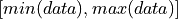.

See the computeBandwidth method for the bandwidth selection.

Examples

Create an histogram:

>>> import openturns as ot
>>> sample = ot.Normal().getSample(50)
>>> histogram = ot.HistogramFactory().build(sample)


Create an histogram from a number of bins:

>>> import openturns as ot
>>> sample = ot.Normal().getSample(50)
>>> binNumber = 10
>>> histogram = ot.HistogramFactory().build(sample, binNumber)


Create an histogram from a bandwidth:

>>> import openturns as ot
>>> sample = ot.Normal().getSample(50)
>>> bandwidth = 0.5
>>> histogram = ot.HistogramFactory().build(sample, bandwidth)


Create an histogram from a first value and widths:

>>> import openturns as ot
>>> ot.RandomGenerator.SetSeed(0)
>>> sample = ot.Normal().getSample(50)
>>> first = -4
>>> width = ot.Point(7, 1.)
>>> histogram = ot.HistogramFactory().build(sample, first, width)


Compute bandwidth with default robust estimator:

>>> import openturns as ot
>>> ot.RandomGenerator.SetSeed(0)
>>> sample = ot.Normal().getSample(50)
>>> factory = ot.HistogramFactory()
>>> factory.computeBandwidth(sample)
0.8207...


Compute bandwidth with optimal estimator:

>>> import openturns as ot
>>> ot.RandomGenerator.SetSeed(0)
>>> sample = ot.Normal().getSample(50)
>>> factory = ot.HistogramFactory()
>>> factory.computeBandwidth(sample, False)
0.9175...


Methods

 build(*args) Build the distribution. buildAsHistogram(*args) Estimate the distribution as native distribution. buildEstimator(*args) Build the distribution and the parameter distribution. buildFromQuantiles(lowerBound, ...) Build from quantiles. computeBandwidth(sample[, useQuantile]) Compute the bandwidth. Accessor to the bootstrap size. Accessor to the object's name. Accessor to the object's id. Accessor to the object's name. Accessor to the object's shadowed id. Accessor to the object's visibility state. Test if the object is named. Test if the object has a distinguishable name. setBootstrapSize(bootstrapSize) Accessor to the bootstrap size. setName(name) Accessor to the object's name. Accessor to the object's shadowed id. setVisibility(visible) Accessor to the object's visibility state.
__init__(*args)
build(*args)

Build the distribution.

Available usages:

build(sample)

build(param)

Parameters:
sample2-d sequence of float

Sample from which the distribution parameters are estimated.

paramCollection of PointWithDescription

A vector of parameters of the distribution.

Returns:
distDistribution

The built distribution.

buildAsHistogram(*args)

Estimate the distribution as native distribution.

If the sample is constant, the range of the histogram would be zero. In this case, the range is set to be a factor of the Distribution-DefaultCDFEpsilon key of the ResourceMap.

Available usages:

build(sample)

build(sample, binNumber)

build(sample, bandwidth)

build(sample, first, width)

Parameters:
sampleSample

Data

binNumberint

The number of classes.

bandwidthfloat

The width of each class.

firstfloat

The lower bound of the first class.

width1-d sequence of float

The widths of the classes.

Returns:
distributionHistogram

The estimated distribution

buildEstimator(*args)

Build the distribution and the parameter distribution.

Parameters:
sample2-d sequence of float

Sample from which the distribution parameters are estimated.

parametersDistributionParameters

Optional, the parametrization.

Returns:
resDistDistributionFactoryResult

The results.

Notes

According to the way the native parameters of the distribution are estimated, the parameters distribution differs:

• Moments method: the asymptotic parameters distribution is normal and estimated by Bootstrap on the initial data;

• Maximum likelihood method with a regular model: the asymptotic parameters distribution is normal and its covariance matrix is the inverse Fisher information matrix;

• Other methods: the asymptotic parameters distribution is estimated by Bootstrap on the initial data and kernel fitting (see KernelSmoothing).

If another set of parameters is specified, the native parameters distribution is first estimated and the new distribution is determined from it:

• if the native parameters distribution is normal and the transformation regular at the estimated parameters values: the asymptotic parameters distribution is normal and its covariance matrix determined from the inverse Fisher information matrix of the native parameters and the transformation;

• in the other cases, the asymptotic parameters distribution is estimated by Bootstrap on the initial data and kernel fitting.

buildFromQuantiles(lowerBound, probabilities, quantiles)

Build from quantiles.

We consider an histogram distribution with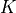bins. Given a set of probabilities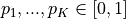and a set of quantiles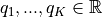, we compute the parameters of the distribution such that: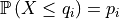for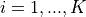.

Parameters:
lowerBoundfloat

Lower bound

probabilitiessequence of float

The probabilities

quantilessequence of float

Quantiles of the desired distribution.

Returns:
distHistogram

Estimated distribution.

Examples

>>> import openturns as ot
>>> ref_dist = ot.Normal()
>>> lowerBound = -3.0
>>> N = 10
>>> probabilities = [(i+1) / N for i in range(N)]
>>> quantiles = [ref_dist.computeQuantile(pi) for pi in probabilities]
>>> factory = ot.HistogramFactory()
>>> dist = factory.buildFromQuantiles(lowerBound, probabilities, quantiles)

computeBandwidth(sample, useQuantile=True)

Compute the bandwidth.

The bandwidth of the histogram is based on the asymptotic mean integrated squared error (AMISE).

When useQuantile is True (the default), the bandwidth is based on the quantiles of the sample. For any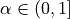, let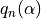be the empirical quantile at level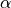of the sample. Let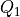and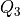be the first and last quartiles of the sample: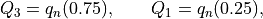and let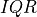be the inter-quartiles range: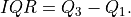In this case, the bandwidth is the robust estimator of the AMISE-optimal bandwidth, known as Freedman and Diaconis rule [freedman1981]: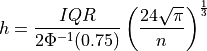where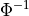is the quantile function of the gaussian standard distribution. The expression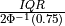is the normalized inter-quartile range and is equal to the standard deviation of the gaussian distribution. The normalized inter-quartile range is a robust estimator of the scale of the distribution (see [wand1994], page 60).

When useQuantile is False, the bandwidth is the AMISE-optimal one, known as Scott’s rule: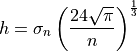where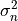is the unbiaised variance of the data. This estimator is optimal for the gaussian distribution (see [scott1992]). In this case, the AMISE is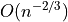.

If the bandwidth is computed as zero (for example, if the sample is constant), then the Distribution-DefaultQuantileEpsilon key of the ResourceMap is used instead.

Parameters:
sampleSample

Data

Returns:
bandwidthfloat

The estimated bandwidth

useQuantilebool, optional (default=True)

If True, then use the robust bandwidth estimator based on Freedman and Diaconis rule. Otherwise, use the optimal bandwidth estimator based on Scott’s rule.

getBootstrapSize()

Accessor to the bootstrap size.

Returns:
sizeinteger

Size of the bootstrap.

getClassName()

Accessor to the object’s name.

Returns:
class_namestr

The object class name (object.__class__.__name__).

getId()

Accessor to the object’s id.

Returns:
idint

Internal unique identifier.

getName()

Accessor to the object’s name.

Returns:
namestr

The name of the object.

Accessor to the object’s shadowed id.

Returns:
idint

Internal unique identifier.

getVisibility()

Accessor to the object’s visibility state.

Returns:
visiblebool

Visibility flag.

hasName()

Test if the object is named.

Returns:
hasNamebool

True if the name is not empty.

hasVisibleName()

Test if the object has a distinguishable name.

Returns:
hasVisibleNamebool

True if the name is not empty and not the default one.

setBootstrapSize(bootstrapSize)

Accessor to the bootstrap size.

Parameters:
sizeinteger

Size of the bootstrap.

setName(name)

Accessor to the object’s name.

Parameters:
namestr

The name of the object.

Accessor to the object’s shadowed id.

Parameters:
idint

Internal unique identifier.

setVisibility(visible)

Accessor to the object’s visibility state.

Parameters:
visiblebool

Visibility flag.

## Examples using the class¶Draw an histogram

Draw an histogramCompare unconditional and conditional histograms

Compare unconditional and conditional histogramsDefine a distribution from quantiles

Define a distribution from quantilesFit an extreme value distribution

Fit an extreme value distributionKolmogorov-Smirnov : get the statistics distribution

Kolmogorov-Smirnov : get the statistics distributionGenerate random variates by inverting the CDF

Generate random variates by inverting the CDFQuick start guide to distributions

Quick start guide to distributionsAggregate processes

Aggregate processesUse the Box-Cox transformation

Use the Box-Cox transformationCreate a polynomial chaos for the Ishigami function: a quick start guide to polynomial chaos

Create a polynomial chaos for the Ishigami function: a quick start guide to polynomial chaosKriging : cantilever beam model

Kriging : cantilever beam modelKriging the cantilever beam model using HMAT

Kriging the cantilever beam model using HMATEstimate a probability with Monte-Carlo on axial stressed beam: a quick start guide to reliability

Estimate a probability with Monte-Carlo on axial stressed beam: a quick start guide to reliabilityEstimate Sobol’ indices for the Ishigami function by a sampling method: a quick start guide to sensitivity analysis

Estimate Sobol' indices for the Ishigami function by a sampling method: a quick start guide to sensitivity analysisA quick start guide to graphs

A quick start guide to graphs